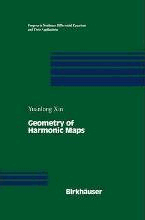# Geometry of Harmonic Maps by Xin, Yuanlong

• R 700.00
Unit price per
• Save R 1,452.49
Tax included.

ISBN: 9780817638207

BOSTON INC | 1 June 1996

Hardback | 246 pages

Harmonic maps are solutions to a natural geometrical variational prob lem. This notion grew out of essential notions in differential geometry, such as geodesics, minimal surfaces and harmonic functions. Harmonic maps are also closely related to holomorphic maps in several complex variables, to the theory of stochastic processes, to nonlinear field theory in theoretical physics, and to the theory of liquid crystals in materials science. During the past thirty years this subject has been developed extensively. The monograph is by no means intended to give a complete description of the theory of harmonic maps. For example, the book excludes a large part of the theory of harmonic maps from 2-dimensional domains, where the methods are quite different from those discussed here. The first chapter consists of introductory material. Several equivalent definitions of harmonic maps are described, and interesting examples are presented. Various important properties and formulas are derived. Among them are Bochner-type formula for the energy density and the second varia tional formula. This chapter serves not only as a basis for the later chapters, but also as a brief introduction to the theory. Chapter 2 is devoted to the conservation law of harmonic maps. Em phasis is placed on applications of conservation law to the mono tonicity formula and Liouville-type theorems.